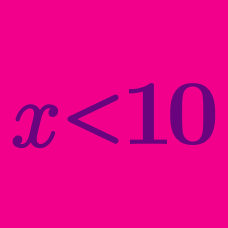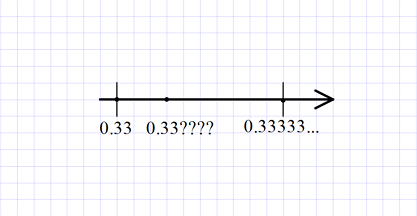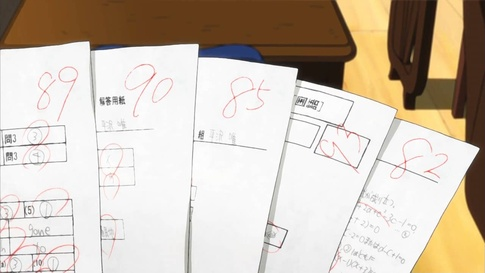Algebra

# Linear Inequalities: Level 3 Challenges

If $A$ is not equal to $B$, which of the following is greater?

$\text{ First: } \frac{ A } {A-B} \quad \quad \text{ Second: } \frac{ B}{A-B}$

Let $a, b, c, d,$ and $e$ be real numbers such that

$a+b

$b+c

$c+d

$d+e.

Which among $a,b,c,d,e$ is the largest and smallest?

Put your answer in the form (largest, smallest). For example, if you think the largest is $c$ and the smallest is $e$, then your answer should be $(c, e)$.Verna decided to sell her collection of books.

To Fred, she sold 2 books, and one fifth of what was left.

Later to Joan she sold 6 books, and one fifth of what then remained.

If she sold more books to Fred than to Joan, what was the least possible number of books in her original collection?$0.33 < \dfrac {m}{n} < \dfrac {1}{3}$

Find the smallest positive integer $n$ such that there exists an integer $m$ for which the inequality above is fulfilled.Mary and three other students took a math test. Each of their scores was a non-negative integer. The teacher announced that the average score (of the 4 students) was 20. Mary immediately knew that all of the other three students scored below average.

What is the minimum score that Mary could have gotten to be certain of the above situation?

×